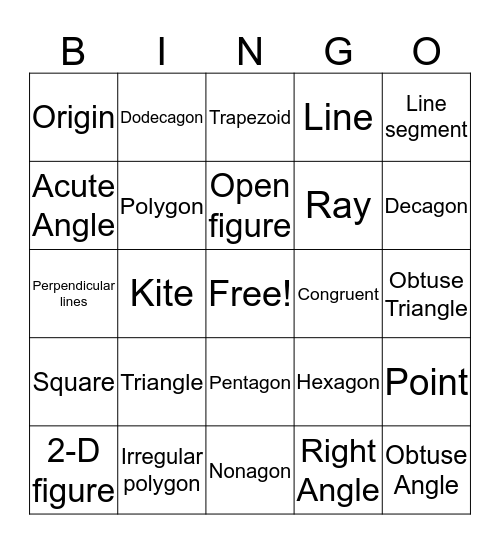# Geometry BingoThis bingo card has a free space and 29 words: 2-D figure, Right Triangle, Kite, Right Trapezoid, Origin, Trapezoid, Polygon, Triangle, Congruent, Irregular polygon, Obtuse Triangle, Obtuse Angle, Nonagon, Right Angle, Decagon, Parallel lines, Pentagon, Square, Line segment, Perpendicular lines, Hexagon, Scalene triangle, Ray, Acute Angle, Line, Dodecagon, Point, Open figure and Closed Figure.

## Play Online